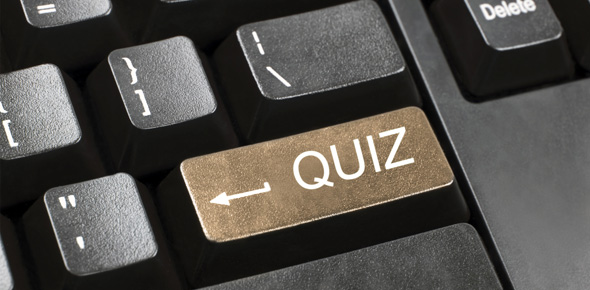Excel Vocabulary Test - Fall 2014

13 Questions | Total Attempts: 168SettingsRead and answer each question carefully. There are different kinds of questions; matching, multiple choices, and true/false.

• 1.
The coordinates of the intersection of a row and column is the ______________.
• A.

Positive reference

• B.

Cell reference

• C.

Name box

• D.

Fill handle

• 2.
The cell into which you are currently entering data; it has a heavy border around it
• A.

Name box

• B.

Cell

• C.

Active cell

• D.

Gridlines

• 3.
The small green square located in the lower right corner of the active cell
• A.

Option handle

• B.

Fill handle

• C.

Status bar

• D.

Range

• 4.
The blinking vertical line that indicates where the next typed character will appear
• A.

Insertion point

• B.

Active cell

• C.

Cell reference

• D.

Fill line

• 5.
The ________________ allows you to add all the numbers in a range of cells.
• A.

MIN function

• B.

SUM function

• C.

MAX function

• D.

• 6.
• A.

• B.

Office Button

• C.

• D.

Name Box

• 7.
The numbers on the left side of the grid are
• A.

• B.

• C.

• D.

Footers

• 8.
The Name Box displays the active cell reference.
• A.

True

• B.

False

• 9.
How are columns designated in Excel?
• A.

Letters

• B.

Numbers

• C.

Triangles

• D.

Combination of letters and numbers

• 10.
This means the cell's content is positioned at the far left in the cell
• A.

Justified

• B.

Right-aligned

• C.

Center-aligned

• D.

Left-aligned

• 11.
The basic unit of a worksheet into which you enter data is called a __________________.
• A.

Cell

• B.

Box

• C.

Range

• D.

GridlineBack to top